System of equations - math word problems - page 27

1. Tractors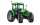Wheel and belt tractors each day plow 8 ha of fields. For plow 76 hectares, the wheel would have to work for 12 days and 8 days with the belt tractor together. How many hectares do each day of each tractor?
2. Boys to girlsThe ratio of boys to girls in a party is 3:5 . If 6 more boys arrived and 4 girls left the party, the ratio of boys to girls would be 5:6 . How many are in the party originally?
3. Mixing waterThe 30-liter container should we fill with water at 60 degrees Celsius. How many liters of water 80 degrees C hot and how many liters of water 20 degrees Celsius warm we have to mix?
4. The sidesThe sides of a rectangle are in a ratio of 2:3, and its perimeter is 1 1/4 inches. What are the lengths of its side? Draw it.
5. New ratio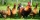The ratio of ducks and chicken in our yard is 2 : 3. The total number of ducks and chickens together is 30. Mother gave 3 of the chickens to our neighbor. What is the new ratio now?
6. Barrel with water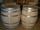The water barrel has a weight of 52 kg. When we pour a quarter of the water, the weight of the barrel is reduced to 40 kg. Determine the weight of the empty barrel.
7. Square into three rectangles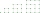Divide the square with a side length of 12 cm into three rectangles with have the same circumference so that these circumferences are as small as possible.
8. Beds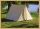At the summer camp, there are 41 chalets. Some rooms are 3-beds, some 4-beds. How many campers from 140 are living in 3-bed?
9. IntegerFind the integer whose distance on the numerical axis from number 1 is two times smaller as the distance from number 6.
10. Simple equationsSolve system of equations: 5x+3y=5 5x+7y=25
11. Company and employeesThere are 370 employees in the company - women are 15% less than men. How many men work in the company?
12. Father and sonWhen I was 11, my father was 35 years old. Today, my father has three times more years than me. How old is she?
13. A candleA candle shop sells scented candles for \$16 each and unscented candles for \$10 each. The shop sells 28 candles today and makes \$400. a. Write a system of linear equations that represents the situation. b. Solve the system to answer the questions: How m
14. Sheep and cowsThere are only sheep and cows on the farm. Sheep is eight more than cows. The number of cows is half the number of sheep. How many animals live on the farm?
15. 13 ticketsA short and long sightseeing tour is possible at the castle. Ticket for a short sightseeing circuit costs CZK 60, for a long touring circuit costs CZK 100. So far, 13 tickets have been sold for 1140 CZK. How much did you pay for tickets for a short tour?
16. Grandfather and grandmotherThe old mother is 5 years younger than the old father. Together they are 153 years old. How many years has each of them?
17. The touristThe tourist traveled 190km in 5 hours. Part of the journey passed at 5 km/h. The rest he went by bus at a speed of 60 km/h. How long has a bus gone?
18. Three sidesSide b is 2 cm longer than side c, side a is 9 cm shorter than side b. The triangle circumference is 40 cm. Find the length of sides a, b, c . .. .
19. Two trainsThere were 159 freight wagons on the railway station creating 2 trains. One had 15 more wagons than the other. How many wagons did each train have?
20. Substitutionsolve equations by substitution: x+y= 11 y=5x-25

Do you have an interesting mathematical word problem that you can't solve it? Enter it, and we can try to solve it.

To this e-mail address, we will reply solution; solved examples are also published here. Please enter e-mail correctly and check whether you don't have a full mailbox.

Do you have a system of equations and looking for calculator system of linear equations?